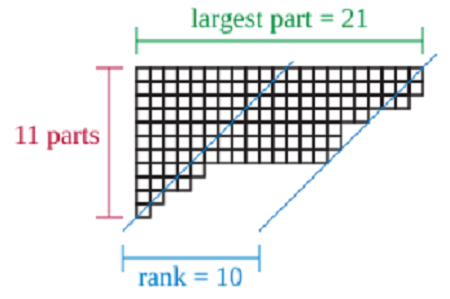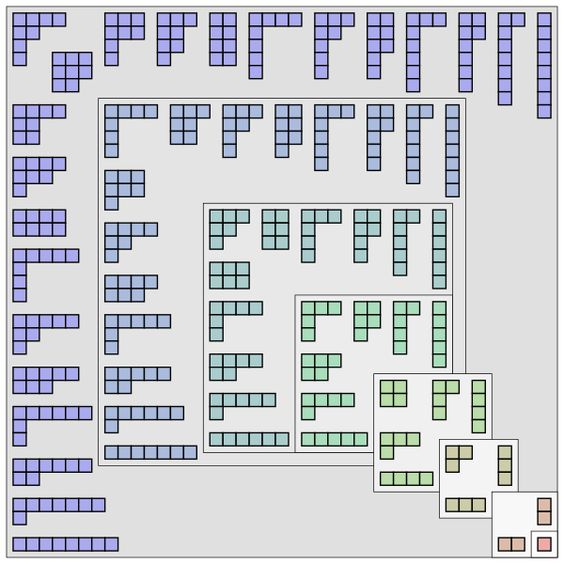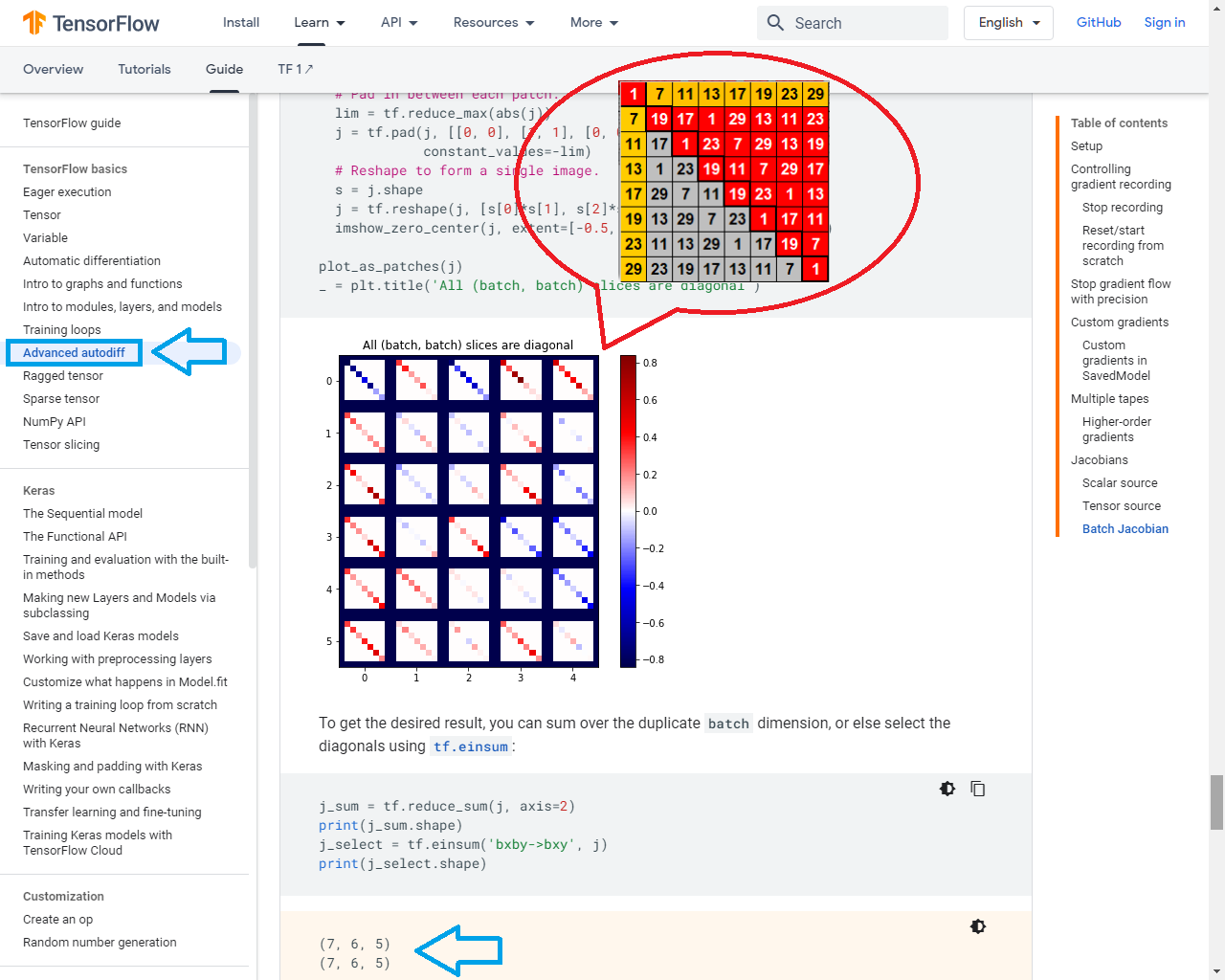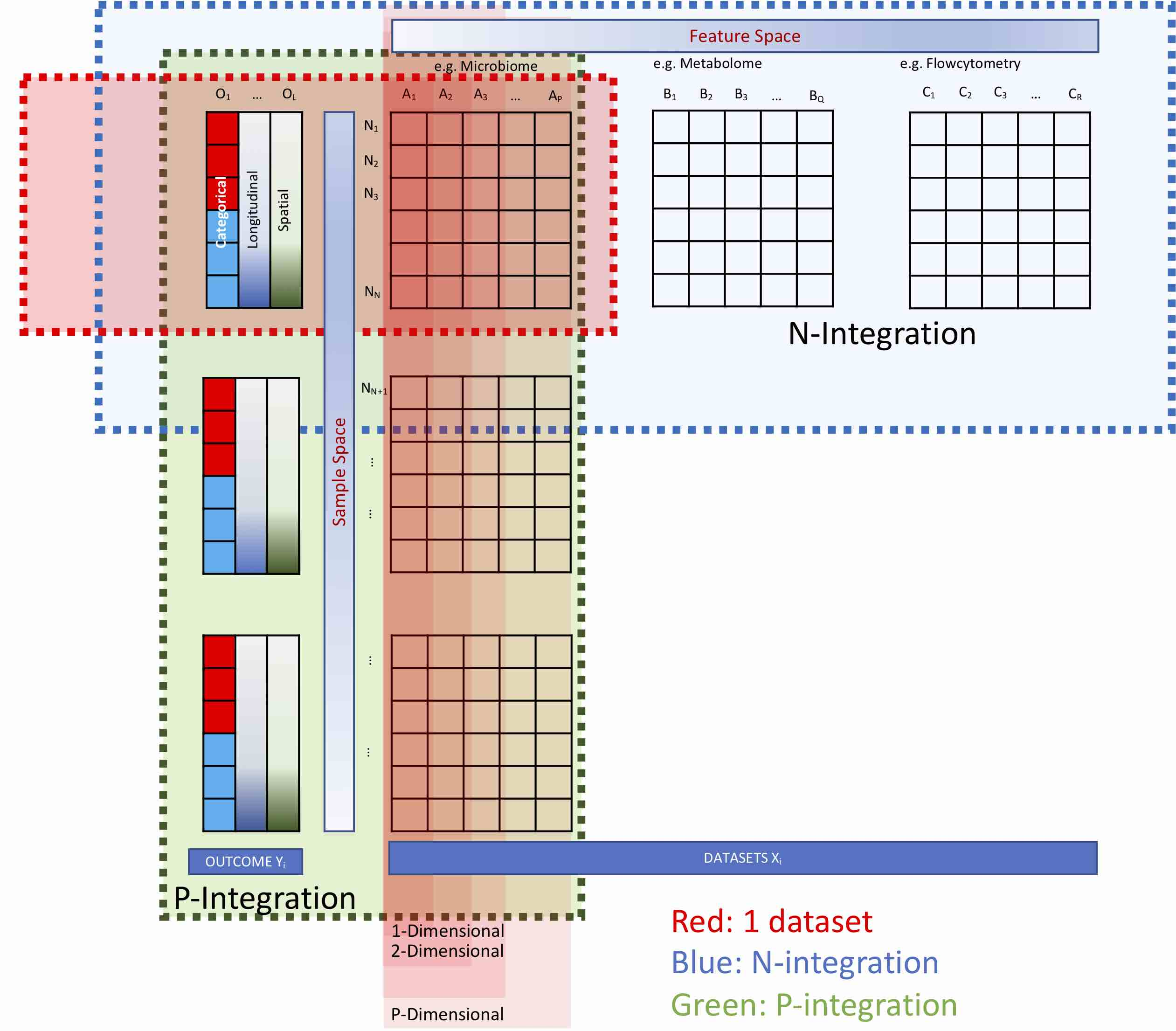# Primes Platform

Prime hexagon is a mathematical structure developed by mathematician T. Gallion that is formed when integers are sequentially added to a field of tessellating equilateral triangles, where the path of the integers is changed whenever a prime is encountered.

This is not easy as they are linked to the nature of prime numbers, and nothing is easy about the nature of prime numbers. But I begin with this assumption: if the hexagons participate in the Universe in any way other than haphazardly, they must be demonstrably congruent to something organized (T. Gallion).This behaviour in a fundamental causal relation to the primes when the products are entered into the partitions system. The subclasses of partitions systemically develops characters similar to the distribution of prime numbers.In number theory, the partition functionp(n) represents the number of possible partitions of a non-negative integer n. Integers can be considered either in themselves or as solutions to equations (Diophantine geometry).

Young diagrams associated to the partitions of the positive integers 1 through 8. They are arranged so that images under the reflection about the main diagonal of the square are conjugate partitions (Wikipedia).The mapping of this sequence will use OOP between 168=π(1000) as lexer and 618=1000/Φ as parser while the 109 objects will be assigned as the runner to automate the workflow.When these subclasses of partitions are flatten out into a matrix, you want to take the Jacobian of each of a stack of targets with respect to a stack of sources, where the Jacobians for each target-source pair are independent .

It's possible to build a Hessian matrix for a Newton's method step using the Jacobian method. You would first flatten out its axes into a matrix, and flatten out the gradient into a vector (Tensorflow).The code is interspersed with python, shell, perl, also demonstrates how multiple languages can be integrated seamlessly. These include generating variants of their abundance profile, assigning taxonomy and finally generating a rooted phylogenetic tree.By taking a distinc function between f(π) as P vs f(i) as NP where e + 1 = 0 then theoretically they shall be correlated to get an expression of the prime platform similar to the Mathematical Elementary Cell 30 (MEC30).